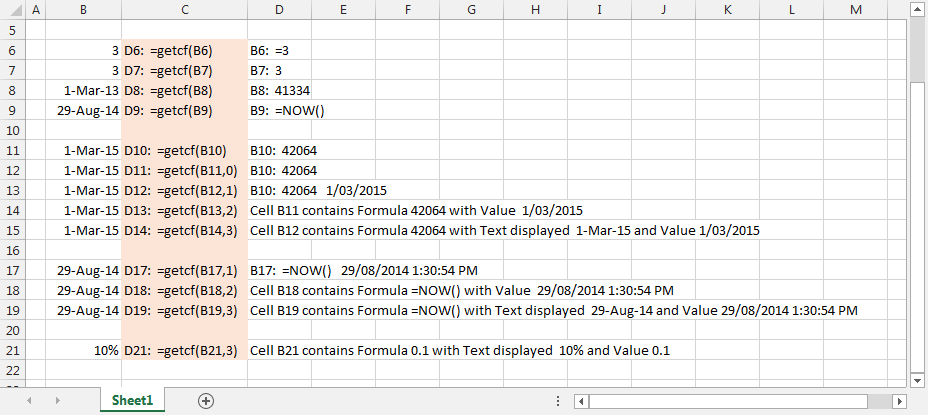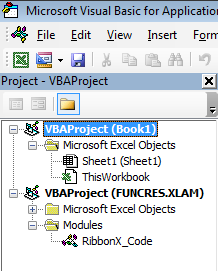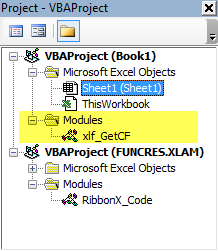# xlf | GetCF function

## 1. GetCF - about the function

Description: returns the formula or text of the given reference as text.

Syntax: GetCF(Ref, [Function_num]).

Arguments: Ref (required): the cell reference, Function_num (optional) - refer to column C of figure 1 and the Select...Case statement in section 3.Fig 1: GetCF function syntax examples - column C of Sheet1 (Click image for more examples of the function_num parameter)

## 2. GetCF - using the function

To use the GetCF function you must add the function code to a VBA module in your workbook. This can be done by importing the function code file to a workbook VBA module, or doing a copy and paste from the Code 1 window below. If you copy and paste, then select the code area to the right of the green line number divider.

The steps for the file import procedure are explained next.

### VBA import

To import the GetCF code to your workbook:

2. Ensure that Developer tab appears on the Excel ribbon. If it is not visible use the File > Options > Customize Ribbon sequence, then, in the Main Tabs panel on the right of the Customize the Ribbon dialog, tick the Developer item. Click OK.

3. Return to the ribbon, and select Developer > Code > Visual Basic to open the Visual Basic Editor (VBE).

4. In the Project Explorer - ensure that the current workbook is selected - Book 1 in this example, see figure 2. If necessary, double click, to select the VBA project for your workbook.

5.Fig 2: Check the current VBAProject workbook - Book1 in this example
1. From the VBE menu, select File > Import File, then use the Import File dialog to select the xlf_GetCF.bas file. Click Open. This will insert a new module named xlf_GetCF in your workbook VBAProject - see figure 3. You have now finished the import task.

2.Fig 3: A new module - xlf_GetCF - has been added to the VBAProject for the workbook
1. Return to Excel by clicking the Excel icon on the VBA toolbar, shown in the upper left of figure 2.

2. Ensure that you save the workbook with an xlsm file type - Excel Macro-Enabled Workbook.

3. If the function returns a #NAME? error, then check your workbook security settings.

## 3. GetCF - the VBA code

Code 1: Function GetCF returns the formula of the given reference as text
Function GetCF(Ref As Range, Optional Function_num As Variant) As String
'' ============================================
'' Description: Returns the formula of the given reference as text
'' Function: Get the Cell Formula (GetCF)
'' Syntax: GetCF(Ref, [Function_num])
'' Get the Cell Formula (GetCF)
'' Arguments:
''   Ref (required): the cell reference
''   Function_num (optional)
'' Developed by: Ian O'Connor
'' Web site: http://excelatfinance.com
'' email: ioconnor@excelatfinance.com
'' ============================================
Dim Form As String
Dim Val As String
Dim Num As Long
Dim RefS As Long
Dim Txt As String
RefS = Application.ReferenceStyle
On Error GoTo ErrHandler:
If IsMissing(Function_num) Then
Num = 0
Else
Num = Function_num
End If
Form = Ref.Formula
Val = Ref.Value
Txt = Ref.Text
Select Case Num
Case 0  ' Default value
GetCF = Addr & ":  " & Form
Case 1
GetCF = Addr & ":  " & Form & "   " & Val
Case 2
GetCF = "Cell " & Addr & " contains Formula " & Form & " with Value  " & Val
Case 3
GetCF = "Cell " & Addr & " contains Formula " & Form & " with Text displayed  " & Txt & " and Value " & Val
Case Else
GetCF = Form
End Select
If Form = "" Then GetCF = ""
Exit Function
ErrHandler:
End Function


• This example was developed in Excel 2013 Pro 64 bit.
• Published: 29 August 2014
• Revised: Wednesday 15th of August 2018 - 03:13 PM, Pacific Time (PT)